Courses

# Parallel Reaction - Chemical Kinetics Chemistry Notes | EduRev

## Physical Chemistry

Created by: Asf Institute

## Chemistry : Parallel Reaction - Chemical Kinetics Chemistry Notes | EduRev

The document Parallel Reaction - Chemical Kinetics Chemistry Notes | EduRev is a part of the Chemistry Course Physical Chemistry.
All you need of Chemistry at this link: Chemistry

PARALLEL REACTION

Parallel reaction are those reaction in which the reactant can form one of two or more products

Consider the following parallel reaction in which reactant A can form two products B & C.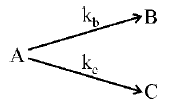The rate law for the reactant and products are :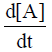= –kB[A] – kc[A] = –(kB + kC)[A]                         …(1)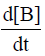= kB[A]                                …(2)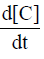= kC[A]                              ....(3)

Integration of equat ion (1) with the init ial condit ion [A]0 ≠ 0 and [B] = 0 = [C] yields

[A] = [A]0 e-(kB + kC )t                         .......(4)

Integration of equation (2), we get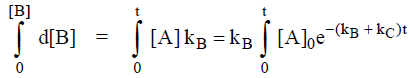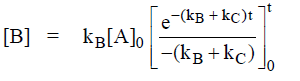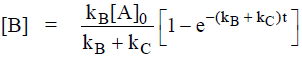…(5)
Similarly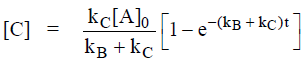…(6)
i.e. the ratio of concentration of product is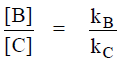i.e. the product concentration ratio remains constant with time.
The yield, φ, is defined as the probability that a given product will be formed by decay of the reactant.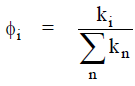The quantum yield of product [B] is φB = yield of [B] =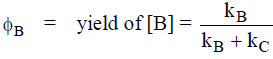The quantum yield of product [C] is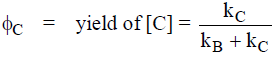Problem.  Find the quantum yield of [B] & [C] in the following reaction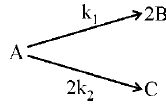Sol.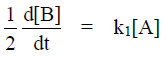…(1)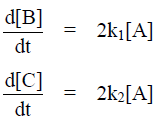…(2)

then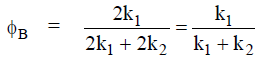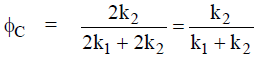Problem.  Find the quantum yield of [B], [C] & [D] in the following reaction.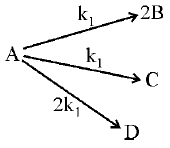Sol.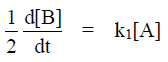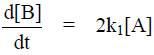…(1)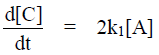…(2)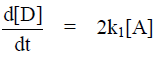…(3)

The ratio of formation of product [B], [C] & [D] are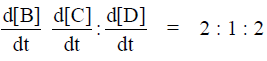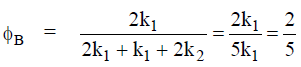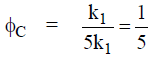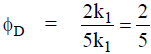Offer running on EduRev: Apply code STAYHOME200 to get INR 200 off on our premium plan EduRev Infinity!

## Physical Chemistry

77 videos|83 docs|32 tests

,

,

,

,

,

,

,

,

,

,

,

,

,

,

,

,

,

,

,

,

,

;